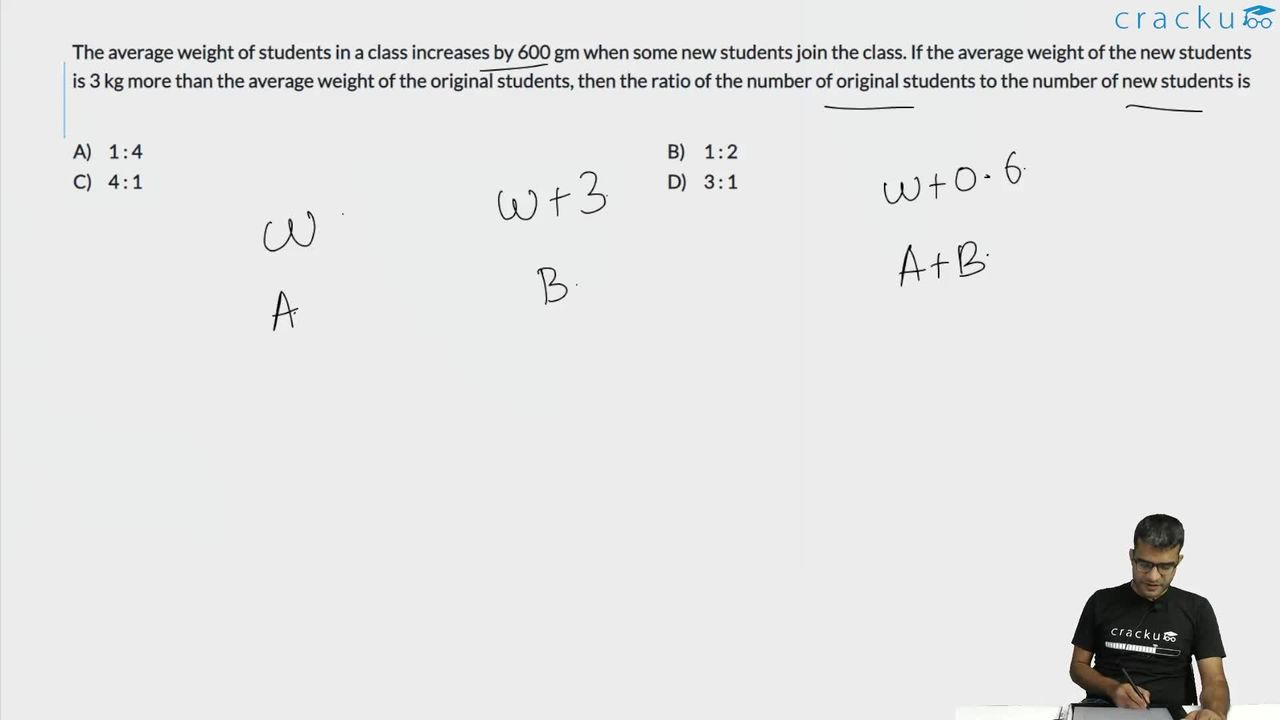Question 46

# The average weight of students in a class increases by 600 gm when some new students join the class. If the average weight of the new students is 3 kg more than the average weight of the original students, then the ratio of the number of original students to the number of new students is

Solution

Let the original number of students be 'n' whose average weight is 'x'

Let the number of students added be 'm' and the average weight will be x + 3

We need to find the value of n : m

It is given, average weight of students in a class increased by 0.6 after new students are added.

Therefore,

$$\ \frac{\ nx+m\left(x+3\right)}{n+m}=x+0.6$$

$$\ \ nx+mx+3m=mx+nx+0.6n+0.6m$$

$$2.4m=0.6n$$

$$4m=n$$

$$\frac{n}{m}=\frac{4}{1}$$

### View Video Solution• All Quant CAT Formulas and shortcuts PDF
• 30+ CAT previous papers with solutions PDF

##### Nischitha

2 months, 3 weeks ago

I'm confused in 4b = a step... How 2.4b=6a become 4b=a??

##### Niits

2 months, 1 week ago

You want to get a/b so bring b below a and take .6 below 2.4
So it will look like a/b = 2.4/.6
a/b = 4/1
Hope it helped.
Drop a thank you or let me know if its still not clear.